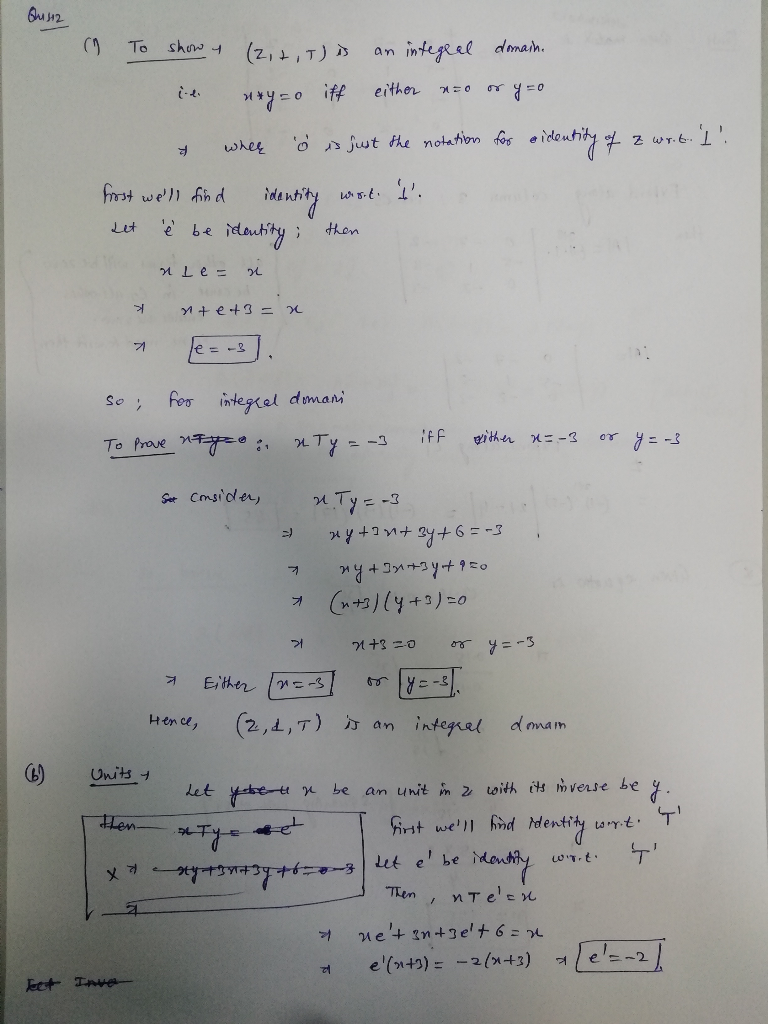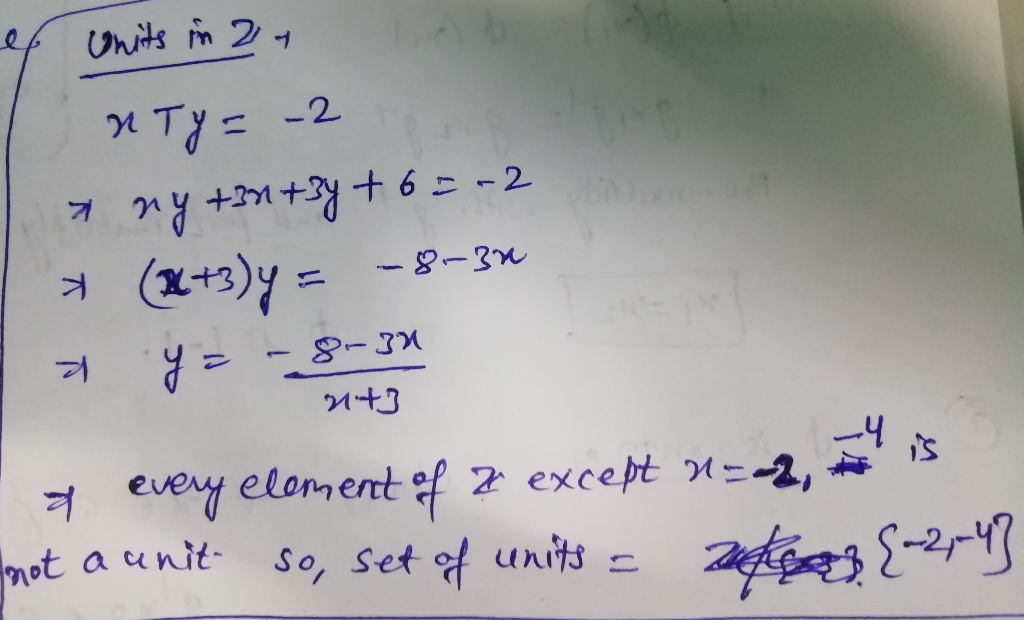Homework Help Question & Answers

2.) Let Z the set of integers and two binary operations on it: Z23(x,y) → xTy = xy + 3x +3y +6 e ...2.) Let Z the set of integers and two binary operations on it: Z23(x,y) → xTy = xy + 3x +3y +6 e Z i) Show (Z,L,T)is an integral domain ii) Find the set of units U(Z)Add Answer of: 2.) Let Z the set of integers and two binary operations on it: Z23(x,y) → xTy = xy + 3x +3y +6 e ...
More Homework Help Questions Additional questions in this topic.

• Additional problem 1 Let AABC be a triangle, let be the bisector of the angle ZCAB Let P be the intersection of and BC. Let R be the point on the line AB such that AR-AC, and let X-APnRC. Let Q denot...

Need Online Homework Help?

Get FREE EXPERT Answers
WITHIN MINUTES
Related Questions